Courses

# Dielectrics (Part - 2) Electrical Engineering (EE) Notes | EduRev

## Electrical Engineering (EE) : Dielectrics (Part - 2) Electrical Engineering (EE) Notes | EduRev

The document Dielectrics (Part - 2) Electrical Engineering (EE) Notes | EduRev is a part of the Electrical Engineering (EE) Course Electromagnetic Theory.
All you need of Electrical Engineering (EE) at this link: Electrical Engineering (EE)

In the previous lecture we obtained a multipole expansion of the potential due to a continuous charge distribution. We discussed in some detail, the dipole part of this expansion and will be doing so in further detail later in this lecture. Let us make some comments on the quadrupoleterm.

The most elementary quadrupole consists of two dipoles oriented anti-parallel. A linear dipole is one where the four charges are lined up with the two dipoles being end to end but directed oppositely, so that there is a charge 2q at the centre and –q each at the ends.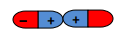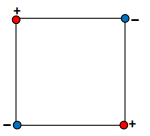Recall that the expression for the quadrupole contribution to the potential is given by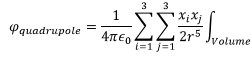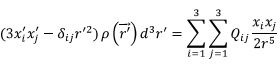where the quadrupole moment tensor is given by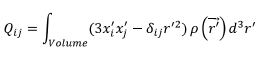Note that since I and j take three values each, the quadrupole moment is a tensor of rank two with 9 components. A few properties of quadrupoleare :

1. The tensor is traceless. (Recall that the trace of a matrix is sum of its diagonal components. This can be easily proved by taking i=j and summing over i.

2. At large distances quadrupolefield decreases as inverse fourth power of the distance from the source. (This is easily proved by inspection as the potential goes as inverse cube of distance).

We now return back to the dipole term which is the most important term for the case of a neutral dielectric. Observe that the dipole moment defined by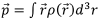depends, in general, on our choice of origin, for, if we let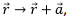we would have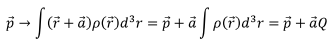Thus only for the case of the dielectric not having a net charge will it be independent of the choice of origin.

We had seen that the potential due to the dipole contribution to the charge distribution is the one that we would obtain if we have a volume charge density and a surface charge density,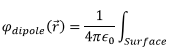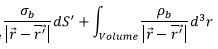where the charge densities are related to the polarization vector by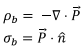It may be emphasized that the bound charges, whether in volume or on the surface, are not fictitious charges; they are very real and their only difference with the free charges which we have in conductors is that they are bound to their parent atoms or molecules.

The “displacement vector”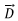is an auxiliary field defined by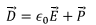Using the relationship of the polarization vector with the bound charge densities, we get,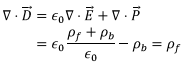Thus the fieldis given by mentally isolating the bound charges from free charges. There is really no physical way of doing it but defining such a displacement vector leads to substantial simplification.

We can easily derive the integral form of the equation satisfied by,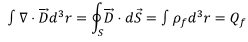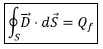where Qf is the total free charges in the sample.

Boundary Conditions on the surface of a dielectric

We recall that in deriving the boundary condition On the surface of a conductor, we had taken a Gaussian Pillbox of infinitesimal height, half inside the conductor And half outside. The field inside the conductor being zero, the contribution to the flux came only from the top face of the pillbox, since the thickness of the pillbox being negligible the curved surface did not contribute. In the present case, there is a difference because the field inside is not zero. Further, one has to remember that at the interface, the normal is outward for the outside face and inward for the inside face.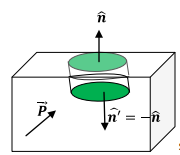Let us assume that there no free charges on the surface.

The volume integral of the electric field over the pillbox is given by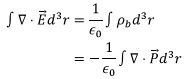Using the divergence theorem on both sides,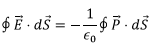The contribution to the surface integral on both sides, as mentioned before, are from the surfaces only with the directions of the normal being opposite,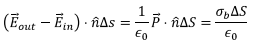Where we have taken the direction of normal in this equation as going away from the surface (i.e. outward). Thus we have,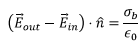This is precisely the relationship that we had earlier for the boundary condition for a conductor except that the surface charge density was due to the free charges. Adding the free charge density, the discontinuity in the normal component of the electric field is given by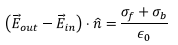We can obtain the discontinuity in the normal component of the displacement vector from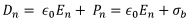We have,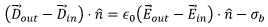the reason for the minus sign is that outside the material σb = 0.

Thus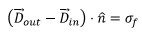Thus, if there are no free surface charges we have the normal component ofto be continuous,

D1n = D2n

The tangential components can be shown to be continuous by use of Stoke’s theorem. We take a rectangular contour across the surface.

E1t = E2t

In the above, the outside medium is denoted as 1 and the inside by 2.

We define a linear dielectric as one where the polarization at a point in the dielectric is proportional to the electric field at the point.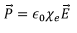The quantity Xe is known as the electric susceptibility. Using the relation between the displacement vector and the electric field vector, we have,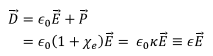The constant k = (1 + Xe) is known as the dielectric constant of the medium and ∈ = ∈0k is known as the permittivity of the medium.

Example 1 : Image Problem with Dielectric

Consider a point charge in a semi-infinite dielectric medium with permittivity ∈1 occupying the space z > 0, the remaining half space z > 0 has a permittivity ∈2. Depending on the problem either of the media could be vacuum with permittivity ∈0.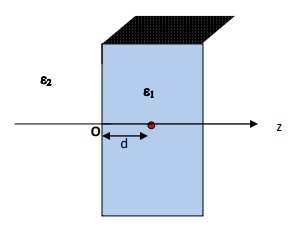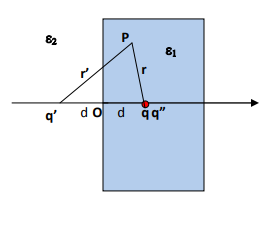The interface is the z=0 plane and has no free charges on it. Based on our experience of solving similar problem using images, we will attempt to write down an expression for the potential at the point P. Since there are no free charges on the surface Dn = Dz is continuous across the surface. Thus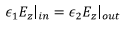In addition, of course, the tangential component of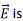continuous. Since the surface is in the x-y plane, we have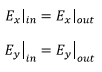In solving this problem, we fall back on the uniqueness theorem and attempt to solve the problem by intuitive method.

Assume that in the medium 1,i.e., in the region z>0, the solution is the same as one would obtain by filling up the entire space by a dielectric of permittivity1 and put an image charge q’  at z = -d. The potential at a point P (the entire space is now a uniform medium) is given by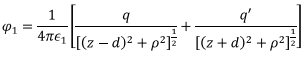Where we have used polar coordinates for the x-y plane, p2 = x2 + y2.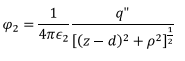For the potential in medium 2, we assume that the solution would be the same as one would obtain by filling up the entire space by a dielectric of permittivit2 and put an image charge at the location of the charge q, i.e. at z = d. We denote the sum of the original charge plus its image by q". Thus the potential at the point P is due to a single charge  q" and is given by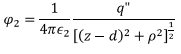Using the continuity of the normal component of the displacement vector,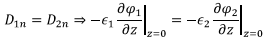(Remember that the direction of the outward normal at the interface was taken as uniquely defined). We have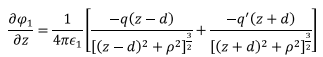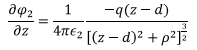Thus we get,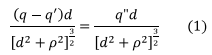The tangential component of the electric field is obtained by differentiating the expressions for the potential with respect to x or y,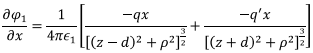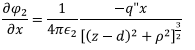So that we have,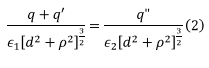From (1) and (2), we obtain,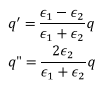In both media Laplace’s equation and the boundary conditions are satisfied and the solution is unique.

Tutorial Assignment

1. Find the first few multipole moments of a linear quadrupole aligned along the z direction.

2. Consider a quadrupole in the xy plane as shown. Determine the components of the quadrupole moment tensor in Cartesian coordinates.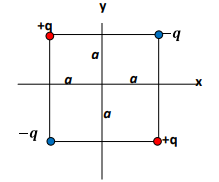3. Two dielectrics having permittivity ∈1 and ∈2 have an interface which has no free charges. The electric field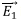in medium 1 makes an angle θ1 with the interface while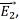the field in medium 2 make an angle θ2. Find the relationship between the two angles.

Solutions to Tutorial assignment

1. In spherical polar coordinates, the charges +q are at (a,0,0) and (a,π,0). The charge densities of the distribution of charges is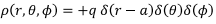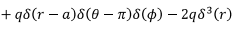The multipole moment of this charge distribution is (for l > 0,)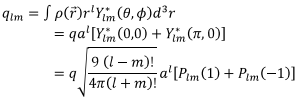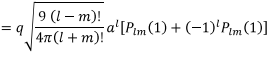The multipole moment vanishes for all odd l. For even l,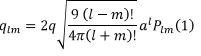Further, plm (1) = 0 except for m=0. Thus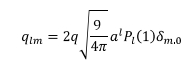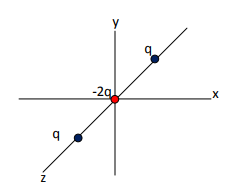(The above formula is valid for even values of l > 0, for l = 0, q00 = 0 because the total charge of the quadrupole is zero)

2. The charge density can be written in terms of delta functions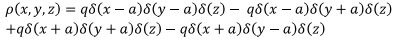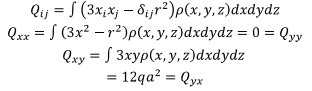All other components are zero because the quadrupole is in the xy plane.

3. At the interface between two dielectrics, the tangential component of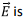continuous

E1 cos θ1 = E2 cos θ2

In the absence of free surface charge at the interface the normal component ofis continuous,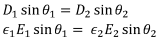Thus, ∈1 tan θ1 = ∈2 tan θ2.

Self Assessment Quiz

1. Find the first few multipole moments of a linear quadrupole aligned along the x direction and also when it is aligned along y direction.

2. Three linear quadrupoles are arranged along the x, y and z axes with the central charge of each being at the origin and the other two charges at a distance a each from the origin. Show that the quadrupole moment of this superposition of the quadrupoles is zero. What is the lowest non-vanishing multipole of this configuration?

3. A rectangular parallelepiped having dimension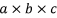is filled with a dielectric in which the polarization is given by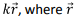is the position vector of a point in the dielectric with respect to the centre of the parallelepiped. Find the bound charge densities in the dielectric and show that the total bound charge is zero.

4. A line charge with a linear charge density λ is in the vacuum, at a distance d from the surface of a semi-infinite dielectric of permittivity ∈. Obtain the image charge that must be put at a distance d inside the dielectric to simulate the charge density at the boundary when the entire space has permittivity ∈0.

5. At the interface between two dielectrics with relative permittivity 2 and 3, there is a free charge density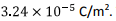The electric field strength in the first medium has a magnitude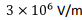and its direction makes an angle of tan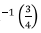with the interface. Find the electric field in the second medium.

Solutions to Self Assessment Quiz

1. The method is the same as that adopted for Problem 1 of the tutorial assignment. When the charges are along the x-axis, the coordinates of the charges +q are at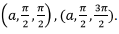The multipole moments are given by, for l > 0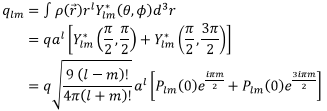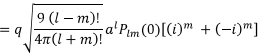R.h.s. is zero for odd values of m. For the quadrupole located along the y axis,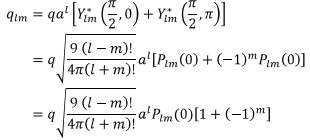Once again, the multipole moment is zero for odd values of m.

2. To find the resulting multipole moments, we add the contribution to the multipole moment from the three cases (Problem 1 of Tutorial and Problem 1 of the Quiz) ,For l > 0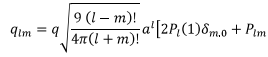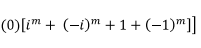We need to only compute even m.
Take l = 1, i.e. the dipole moment. For l = 1, m = 0. Recall that the first term is zero for odd value of l. Further since p10(0) = 0, the dipole moment also vanishes.

Let us compute quadrupole moments for which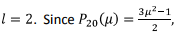We have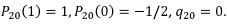For calculating q22 note that the first term is zero because of Kronecker delta function while the second term valishes. Thus all components of the quadrupole moment vanishes .

The next moment is for l = 3. The first term only contributes for even l, and, for the second term we have  p3m (1) = 0. Thus the octupole moments also vanish. The first non-zero moment is l = 4, which is called” hexadecupole” moment.

3. Let us position the dielectric so that the end faces are parallel to the three Cartesian axes.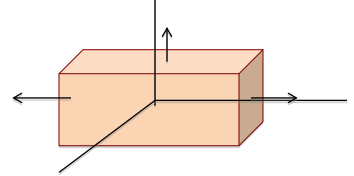The volume density of bound charges is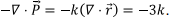Since the density is constant, the total volume charge is obtained by multiplying this with the volume, i.e., qb = -3k abc. Now we need to calculate the bound charges on the surface. Note that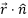on each face is given by the distance of that face from the centre. For instance, the top face (or the bottom face) which is at a distance b from the origin has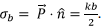The total bound charge on this face is obtained by multiplying the constant density with the area of the face ac giving a contribution of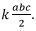Each of the six faces contribute the same, giving a total of which is exactly the equal and opposite of the total volume charge

4. Let the charge density at the interface be . If the entire region had permittivity ∈0, the electric field at the interface can be calculated by taking a Gaussian cylinder perpendicular to the sheet and its ends equidistant from the sheet. The normal component of the electric field (directed outward from the respective face)due to the induced charge is given by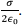Let the normal component of the electric field at the point P on the sheet be due to the line charge be En.

At a point P,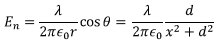Since the normal components are oppositely directed, the resultant normal component is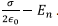The normal component of the net electric field on the other side of the interface is given by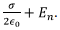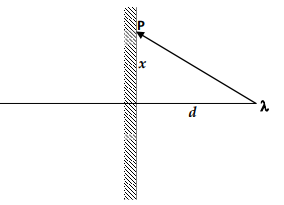Since the normal component of D field is continuous, we have (recall that the outward normal direction is uniquely defined)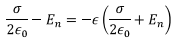Solving, we get,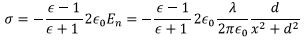This is the induced charge. The field due to this at P is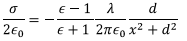Comparing this with the expression for the normal component of the field, we see that this field is equivalently produced by locating an image line with line charge density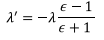at a distance d from the interface, inside the dielectric.

5. At the interface between two dielectrics, the tangential component of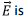continuous E1 cos θ1 = E2 cos θ2. Since the interface has free charges, we have D2 sin θ - D1 sin θ = σf. The last relation gives ∈2 E2 sin θ2 - ∈1E1 sin θ1 = σf.

Thus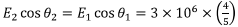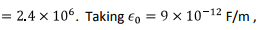we get from the second equation,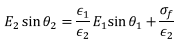Substituting values,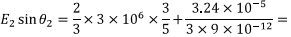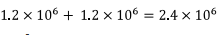Thus tan θ2 = 1 i.e. the electric field makes an angle of 450 with the interface.
The field strength in the second medium is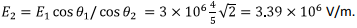Offer running on EduRev: Apply code STAYHOME200 to get INR 200 off on our premium plan EduRev Infinity!

,

,

,

,

,

,

,

,

,

,

,

,

,

,

,

,

,

,

,

,

,

;